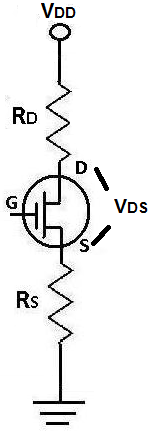﻿ How to Calculate the Drain-Source Voltage, VDS, of a JFET Transistor?# How to Calculate the Drain-Source Voltage VDS of a JFET TransistorThe drain-source voltage, VDS, of a JFET transistor is the voltage that falls across the drain-source terminal of the transistor.

### Calculating the Drain-Source Voltage, VDS

The formula to calculate the drain-source voltage VDS is:

VDS= VD - VS

The only way to calculate VDS is by obtaining the separate voltages, VD and VS. VD is the voltage that is supplied to the drain of the transistor. VS is the voltage that falls across the source of the transistor.